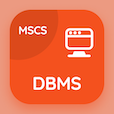Computer Science Online Courses

Digital Logic Design Quizzes

Digital Logic Design Quiz PDF - Complete

# Magnitude Comparator Multiple Choice Questions p. 74

Study Magnitude Comparator multiple choice questions and answers, magnitude comparator quiz answers PDF 74 to study Digital Logic Design course online. MSI and PLD Components MCQ trivia questions, magnitude comparator Multiple Choice Questions (MCQ) for online college degrees. "Magnitude Comparator MCQ" PDF eBook: adder and subtractors, half subtractors, rectangular shape symbols, design procedure of asynchronous sequential logic, magnitude comparator test prep for free online classes.

"A procedure that specifies a finite set of steps is called" MCQ PDF: flow chart, algorithm, chart, and venn diagram for computer majors. Learn msi and pld components questions and answers to improve problem solving skills for best online schools for computer science.

## Magnitude Comparator Questions and Answers MCQs

MCQ: A procedure that specifies a finite set of steps is called

algorithm
flow chart
chart
Venn diagram

MCQ: In the design procedure of the asynchronous circuit, the flow of the table

increased to maximum states
reduced to minimum states
changed
remain same

MCQ: The standard for components recognition is the standard given by

ANSI
ANIS
AISN
AINS

MCQ: The simplified expression of half subtractor borrow is

B=x+y
B=xy
B=x'y
B=xy'

MCQ: Integrated Circuit (IC) 7483 is 4-bit binary

serial subtract or
parallel subtract or

### More Quizzes from Digital Logic Design Course